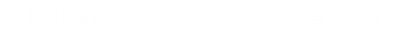# Formulas

Add strings or basic mathematical values in fields and leverage these operands to either perform mathematical equations or concatenate string operands. This tutorial shows the difference between the three.

The Formula form field property lets you define the content of a field based on specific logical operations performed on one or more other text fields. Text or Area Text fields can be used as operands in formulas. Each field is defined as either a String operand or a Number operand, as follows:

• Fields with a validation type of 'Numeric' or 'Numeric decimal' are defined as Number operands.
• Fields with validation types other than 'Numeric' or 'Numeric decimal' are defined as String operands.

#### Supported Operations for String Operands

• Concatenation
• Conversion
• Case Statement
• Regular Expression Parsing
• String Length
• Date to String
• String Replace - Upper/Lower Case

#### Supported Operations for Numerical Operands

• Subtraction
• Multiplication
• Division
• Conversion
• Case Statement
• Random Integer

#### Supported Date Field Formulas

• Date Difference

## Formula Examples

Only 'Text' and 'Area' fields are allowed as operands in formulas expressions.

Each 'Text' field can be defined as String (by default) or Number operand.

Fields with validation types 'Numeric' and 'Numeric decimal' will be defined as  Number operands.

Fields with other validation types will be defined as String operands

Allowed operations for Strings.

 Operations Operators Samples Concatenation + @FORMelement1+@FORMelement2+'  senior manager.' When element1 = 'John', element2 = 'Smith'. Result will be:  'JohnSmith senior manager.' Note: If you need add some constant text to formula expression, add this text with single quotas. If you need add quota, you should add escape character before it: @FORMelement1+'employee\'s' Conversion convert convert(@FORMelement1, 'Int32') + 10 Result will be integer number   convert(@FORMelement1, 'Decimal')  Result will be decimal number   convert(@FORMelement1, 'DateTime') Result will be DateTime   convert('08/16/2017', 'DateTime') Result will be DateTime Case statement case case    when @FORMelement1='NYC' then 'New York'    when @FORMelement1='DC' then 'Washington, D.C'    else @FORMelement1    else ' ' end + ' the best city.' when element1='NYC' result will be 'New York the best city.' when element1='DC' result will be 'Washington, D.C the best city.' when element1='Denver' result will be 'Denver the best city.'   Allowed operations with 'when' statement: '=' - equal  '<>' - not equal Regular Expression Parsing RegExExtract RegExExtract(@FORMelement1,'\-(.*?)\-') If the value entered into FORMelement1 = '123-456-789' the result will be 456 Note: The form element must be the first argument to the function and the single quotes are necessary around the regular expression For assistance building and testing regular expressions: https://regexr.com/ Isdefault() Isdefault() case when isDefault(@FORMelement1) then 'Not Filled' else FORMElement1 + ' ' + FORMElement2 end If a  form consists of two fields, First Name (FORMElement1) and Last Name (FORMelement2)  and we want to create a third field that gets set to First Name + Last Name if first name is having a value otherwise  it will display value Not Filled only. When element1=' '  and element2= ' New York' result will be   'Not Filled' When element1='New' and element2='York'  result will be 'New York' String Length StringLength() StringLength(@FormElement1) When a word is entered, the number of strings in the word is displayed. Change Date to String DateToString() DateToString(@FORMelement1,'dd MMMM yyyy') Date format can be selected from the date picker options. If the date entered is 02/02/2023, the result will be '02 February 2023'. Replace String StringReplace() StringReplace(@FORMelement1,'Test','New-Test') In form element 1, the string 'Test' will be replaced with 'New-Test' and displayed. Even if a prefix or suffix is added, the string is displayed along with it. To Upper case ToUpper() ToUpper(@FORMelement1) The text entered in the field will be displayed in uppercase. To Lower case ToLower() ToLower(@FORMelement1) The text entered in the field will be displayed in lowercase.

Allowed operations for Numbers.

 Operations Operators Samples Addition + @FORMelement1+@FORMelement2 When element1 = '1', element2 = '2'. Result will be: '3' Subtraction - @FORMelement1-@FORMelement2 When element1 = '3', element2 = '2'. Result will be: '1' Multiplication * @FORMelement1*@FORMelement2 When element1 = '3', element2 = '2'. Result will be: '6' Division / @FORMelement1/@FORMelement2 When element1 = '6', element2 = '2'. Result will be: '3'. Note: You will get infinity '∞' in case of division by 0 Conversion convert convert(@FORMelement1+@FORMelement2, 'string') +'  Dalmatians' When element1 = '100', element2 = '1'. Result will be: '101 Dalmatians'. Case statement case case    when @FORMelement1/@FORMelement2 <= 0 then 0   when @FORMelement1/@FORMelement2 >= 100 then 100   else @FORMelement1/@FORMelement2 end when elemen1=1, elemen1=-2 result will be 0 when elemen1=1000, elemen1=2 result will be 100 when elemen1=100, elemen1=2 result will be 50   Allowed operations with 'when' statement: '>' - more '>=' - more or equal '<' - less '<=' less or equal '=' - equal  '<>' - not equal Random Integer integers IntRandInt RandInt(@FORMelement48,FORMelement45) Displays a random integer between two numbers entered.

Date Field Formulas